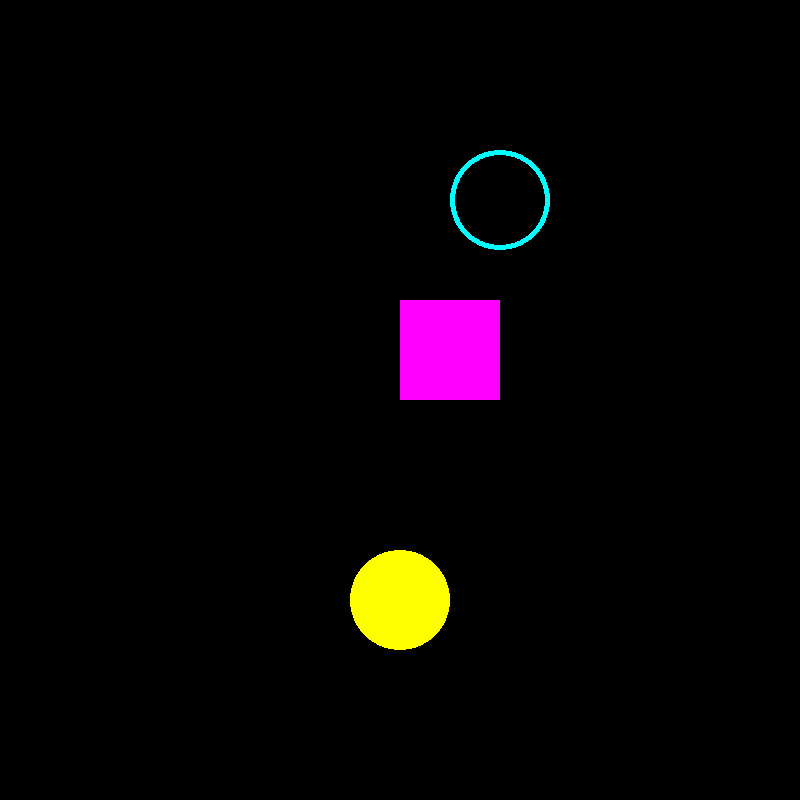# Simple Shapes¶

This example shows how to use the UI API. We will demonstrate how to draw some geometric shapes from DIPY UI elements.

First, a bunch of imports.

```from fury import ui, window
```

Let’s draw some simple shapes. First, a rectangle.

```rect = ui.Rectangle2D(size=(100, 100), position=(400, 400), color=(1, 0, 1))
```

Then we can draw a solid circle, or disk.

```disk = ui.Disk2D(outer_radius=50, center=(400, 200), color=(1, 1, 0))
```

```ring = ui.Disk2D(outer_radius=50, inner_radius=45,
center=(500, 600), color=(0, 1, 1))
```

Now that all the elements have been initialised, we add them to the show manager.

```current_size = (800, 800)
show_manager = window.ShowManager(size=current_size,
title="DIPY Shapes Example")